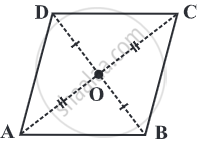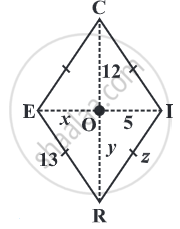# The diagonals of a rhombus are perpendicular bisectors of one another.Given: ABCD is a rhombus.

To Prove: m∠ AOD = m∠ COD = 90°.

Proof:

ABCD is a rhombus.                                                                         .........(Given)

Since the opposite sides of a rhombus have the same length, it is also a parallelogram.  ........(Properties of a rhombus)

The diagonals of a rhombus bisect each other.                            ..........(Properties of a rhombus)

Thus,

OA = OC            .....(DB ⟂ AC, Divides AO and OC into two equal parts)(1)

OB = OD            ......(AC ⟂ DB, Divides DO and OB into two equal parts)(2)

In ∆AOD and ∆COD,
OA = OC             .....(From 1)
OB = OD             ......(From 2)
AD = CD             ......(All the sides of a rhombus are equal.)

by SSS congruency criterion,
∆ AOD ≅ ∆ COD

Therefore, m∠ AOD = m ∠ COD.......(C.A.C.T.)

Since, ∠AOD and ∠ COD are a linear pair.

m∠ AOD = m∠ COD = 90°.

Hence Proved.

#### Example

RICE is a rhombus. Find x, y, z. Justify your findings.x = OE = OI = 5       .....(diagonals bisect)
y = OR = OC = 12.  .....(diagonals bisect)
z = side of the rhombus = 13 .......(all sides are equal).
If you would like to contribute notes or other learning material, please submit them using the button below.

### Shaalaa.com

To Prove that the Diagonals of a Rhombus Bisect Each Other at Right Angles [00:14:21]
S
0%